# Supplementary angles

One of the supplementary angles is larger by 33° than the second one. Calculate the angles size.

A =  106.5
B =  73.5

### Step-by-step explanation:

A+B = 180
A = 33+B

A+B = 180
A-B = 33

A = 213/2 = 106.5
B = 147/2 = 73.5

Our linear equations calculator calculates it.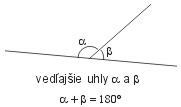Did you find an error or inaccuracy? Feel free to write us. Thank you!Tips to related online calculators
Do you have a linear equation or system of equations and looking for its solution? Or do you have a quadratic equation?

## Related math problems and questions:

• Supplementary angles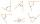One of the supplementary angles are three times larger than the other. What size is larger of supplementary angles?
• MO Z7–I–6 2021In the triangle ABC, point D lies on the AC side and point E on the BC side. The sizes of the angles ABD, BAE, CAE and CBD are 30°, 60°, 20° and 30°, respectively. Find the size of the AED angle.
• Supplementary angles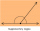(a + 30)° and (2a)° are the measure of two supplementary angles. Find the value of 'a'.
• Angles of a triangleIn triangle ABC, the angle beta is 15° greater than the angle alpha. The remaining angle is 30° greater than the sum of the angles alpha and beta. Calculate the angles of a triangle.
• TrianglesFind out whether given sizes of the angles can be interior angles of a triangle: a) 23°10',84°30',72°20' b) 90°,41°33',48°37' c) 14°51',90°,75°49' d) 58°58',59°59',60°3'
• Internal anglesOne internal angle of the triangle JAR is 25 degrees. The difference is the size of the two others is 15°. Identify the size of these angles.
• Angles in ratioThe size of the angles of the triangle are in ratio x: y = 7: 5 and the angle z is 42° lower than the angle y. Find size of the angles x, y, z.
• Internal anglesThe ABCD is an isosceles trapezoid, which holds: |AB| = 2 |BC| = 2 |CD| = 2 |DA|: On its side BC is a K point such that |BK| = 2 |KC|, on its side CD is the point L such that |CL| = 2 |LD|, and on its side DA the point M is such that | DM | = 2 |MA|. Dete
• Outer anglesThe outer angle of the triangle ABC at the A vertex is 71°40 ' outer angle at the vertex B is 136°50'. What size has the inner triangle angle at the vertex C?
• AnglesThe outer angle of the triangle ABC at the vertex A is 114°12'. The outer angle at the vertex B is 139°18'. What size is the internal angle at the vertex C?
• In a 2In a thirteen sided polygon, the sum of five angles is 1274°, four of the eight angles remaining are equal and the other four are 18° less than each of the equal angles. Find the angles. .
• Angles in triangleThe triangle is ratio of the angles β:γ = 6:8. Angle α is 40° greater than β. What are the size of angles of the triangle?
• The second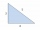The second angle of a triangle is the same size as the first angle. The third angle is 12 degrees larger than the first angle. How large are the angles?
• Inner anglesThe magnitude of the internal angle at the main vertex C of the isosceles triangle ABC is 72°. The line p, parallel to the base of this triangle, divides the triangle into a trapezoid and a smaller triangle. How big are the inner angles of the trapezoid?
• Angle at the apex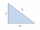In an isosceles triangle, the angle at the apex is 30° greater than the angle at the base. How big are the internal angles?
• Neighbor angle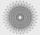For 136° angle calculate size of adjacent angle on one side of a straight line.
• RWYCalculate the opposite direction of the runway 13. Runways are named by a number between 01 and 36, which is generally one tenth of the azimuth of the runway's heading in degrees: a runway numbered 09 points east (90°), runway 18 is south (180°), runway 2